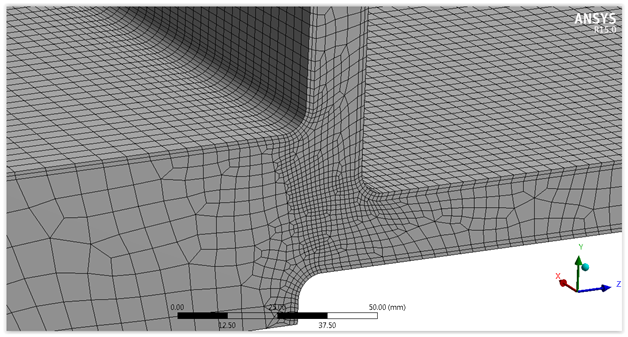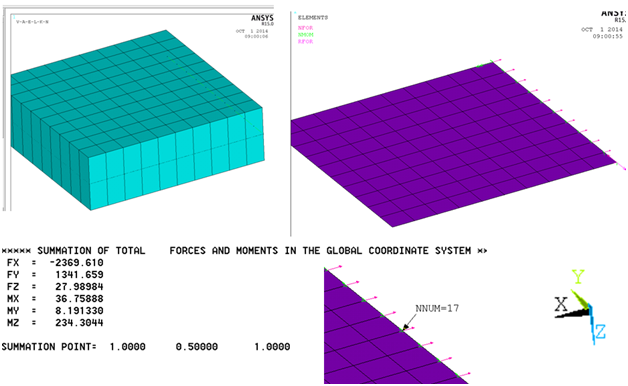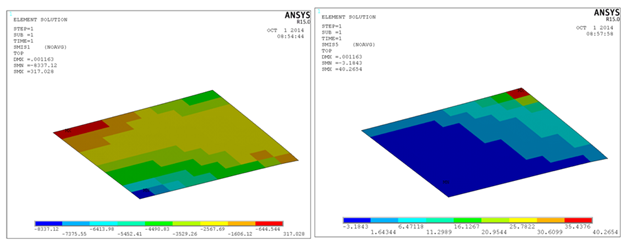you are here:/ News & Insights / Engineering Advantage Blog / Understanding and Using Shell Element Results - Part II

# Understanding and Using Shell Element Results - Part IINovember 28, 2014 By: Peter Barrett

This post is the second in a two-part series that discusses the use of shell elements for finite element analysis. My first post discussed the following questions:

• Are the stresses I plotted real and what are the limitations?
• Are only nominal stress results needed?

If you have not read Part I, you can do so here. I suggest you read it before continuing!

Today, I will be investigating these questions:

• How do I calculate local hot spot stresses needed for fatigue?
• If this a bolted or welded region that I am plotting stresses in, how do I use them?
• When should I use forces and moments instead of stresses?

How do I calculate local hot spot stresses needed for fatigue?

If fatigue life is an issue, then accurate stress results are required. If the high stress region is at a connection and the joint is a casting or machined part for example, then a 3D brick model with detailed fillet radii should be modeled to predict the true stress in the actual connection geometry. Using shell-to-solid submodeling is often the best solution for this type of simulation. Figure 4 illustrates a local 3D model with fillets at the plate interfaces where local detailed stresses could be calculated. An alternative to the local submodel is to apply an empirical stress concentration factor onto the nominal stresses. This factor can come from literature or detailed finite element calculations to account for the local connection geometry.Is this a bolted or welded region that I am plotting stresses in?

If the plate assembly is welded or bolted, then designing the welds or bolts is often better achieved by extracting local forces and moments rather than using stress data.  Figure 5 illustrates forces extracted from the end of the thicker plate at the joint connection. Occasionally, more refined techniques are used for weld stresses. These include:

•  Structural hot spot stress method
•  Effective notch stress method
•  Stress intensity at a crack tip

More details on these techniques can be found here. Similarly, bolted connections can be modeled at various levels of refinement.Do I really need forces and moments instead of stresses?

In many design applications governed by structural codes, stress data is not needed.  Instead, forces and moments are required input; for concrete slab design for example.  The preferred format in this case is in-plane forces and out-of-plane moments typically defined as force or moment per unit length. It is generally preferred to isolate each plate independently and then capture these forces and moments at the element centroids since local hotspots can typically be ignored.  Figure 6 illustrates in-plane axial forces and out-of-plane bending moment results for the bottom left thicker slab. I hope you found this blog useful!  I am curious what shell stress postprocessing methods you can share!